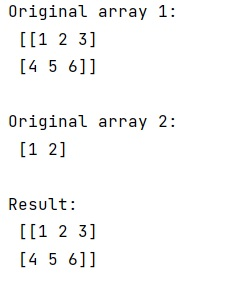NumPy | Loading compressed data from file: In this tutorial, we will learn how to load compressed data (.npz) from file using numpy.load() method in Python? By Pranit Sharma Last updated : April 10, 2023

## Overview

We are given an array and we need to save this array in compressed form (.npz format) which can be done using numpy.savez() function.

However, when we open this compressed file to perform some operations, many a time this data is not accessible and it generates a ValueError. This error can be avoided (explained below).

To load compressed data from file using numpy.load(), pass the file_name, and if the extension is .npz, it will first decompress the file. After that, we need to index the variable in which the decompressed file is stored with the original array to read the data.

Let us understand with the help of an example,

## Python code to load compressed data (.npz) from file using numpy.load()

```# Import numpy
import numpy as np

# Creating arrays to save in compressed form
a=np.array([[1, 2, 3], [4, 5, 6]])
b=np.array([1, 2])

# Display original arrays
print("Original array 1:\n",a,"\n")
print("Original array 2:\n",b,"\n")

# Saving data in compressed form
np.savez('arr.npz', a=a, b=b)

# Display data values of key a
print("Result:\n",data['a'])
```

### Output

```Original array 1:
[[1 2 3]
[4 5 6]]

Original array 2:
[1 2]

Result:
[[1 2 3]
[4 5 6]]
```

### Output Screenshot